# Trigonometric Integrals

 Friday: Quiz 2 Next: Trig subst.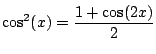and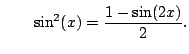(5.2)

Example 5.2.1   Compute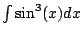.
We use trig. identities and compute the integral directly as follows: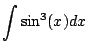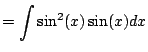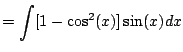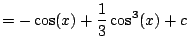(substitution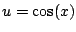)

This always works for odd powers of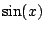.

Example 5.2.2   What about even powers?! Compute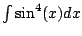. We have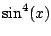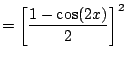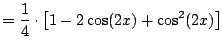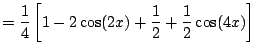Thus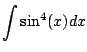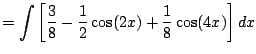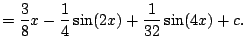Key Trick: Realize that we should writeas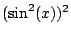. The rest is straightforward.

Example 5.2.3   This example illustrates a method for computing integrals of trig functions that doesn't require knowing any trig identities at all or any tricks. It is very tedious though. We computeusing complex exponentials. We have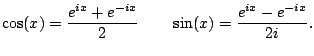hence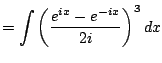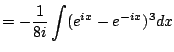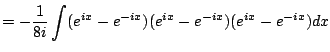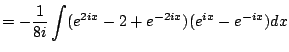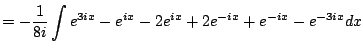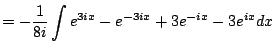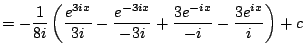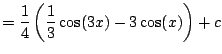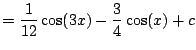The answer looks totally different, but is in fact the same function.

Here are some more identities that we'll use in illustrating some tricks below.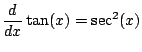and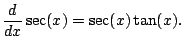Also,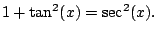Example 5.2.4   Compute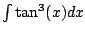. We have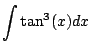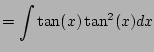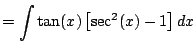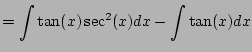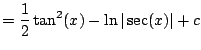Here we used the substitution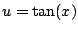, so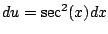, so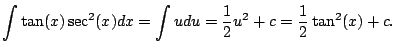Also, with the substitutionand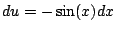we get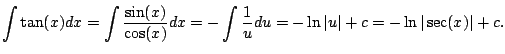Key trick: Write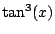as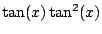.

Example 5.2.5   Here's one that combines trig identities with the funnest variant of integration by parts. Compute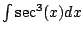.
We have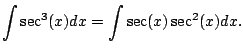Let's use integration by parts.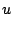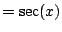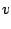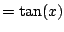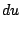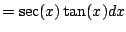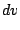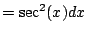The above integral becomes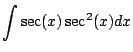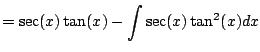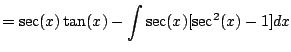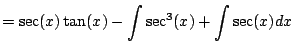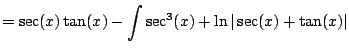This is familiar. Solve for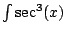. We get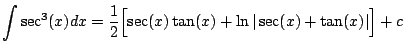Subsections
William Stein 2006-03-15